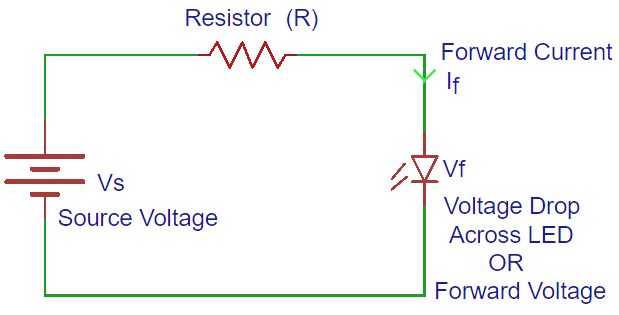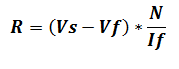# LED Resistor Calculator

Use this LED resistor calculator to find out the suitable resistance for your LED circuit consisting one or more LEDs.

### Inputs

Volt
Volt (Typical value: 2v)
Milliamps (Typical value: 20mA)

Ohms
Watts

### Working of LED Resistor Calculator

Every LED is having a specific range of operating current, beyond the rated level of current it will damage. For the protection or limiting the current we simply use a resistor in series with it.

This LED resistor calculator will help you to pick up a right value of resistor for the LED in your LED circuit, you just have to input the values of Source voltage (Vs), LED forward current (If) and Led forward voltage (Vf).The Forward voltage or voltage drop across the led is predefined (shown in table below), because it depends on the color emit by a LED, typical value of voltage drop is 2v.

 Color Voltage Drop(Vf) Red 2 Green 2.1 Blue 3.6 White 3.6 Yellow 2.1 Orange 2.2 Amber 2.1 Infrared 1.7

### Equation

For finding the value mathematically you can use the equation below:Where,

Vs = Source voltage measured in volts.

Vf = LED forward voltage or voltage drop,if you don’t know the voltage drop of your led then you can use 2v as it is the typical value for an LED’s voltage drop.

If = LED forward current, if you don’t know LED forward current of your led then you can use 20mA as it is the typical value for an LED’s forward current.

N = Number of LEDs to be connected in series.